Question

Help please Two particles, with charges of 20.0 nC and -20.0 nC, are placed at the...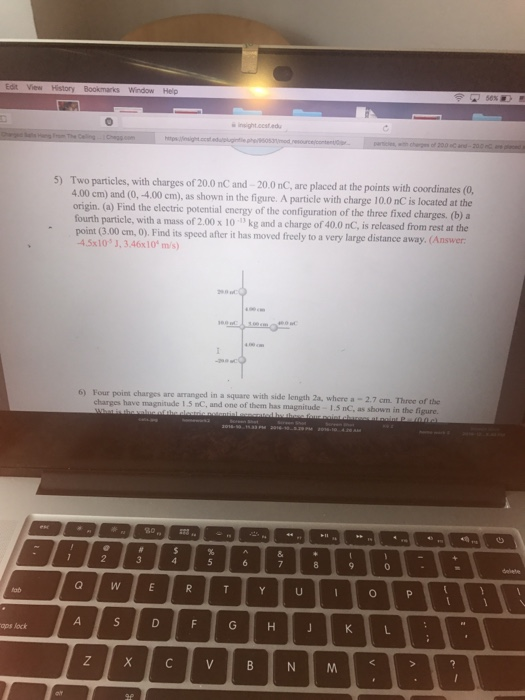Help please
Two particles, with charges of 20.0 nC and -20.0 nC, are placed at the points with coordinates (0, 4.00 cm) and (0, -4.00 cm), as shown in the figure. A particle with charge 10.0 nC is located at the origin. Find the electric potential energy of the configuration of the three fixed charges. a fourth particle, with a mass of 2.00 times 10^-13 kg and a charge of 40.0 nC, is released from rest at the point (3.00 cm, 0), Find its speed after it has moved freely to a very large distance away.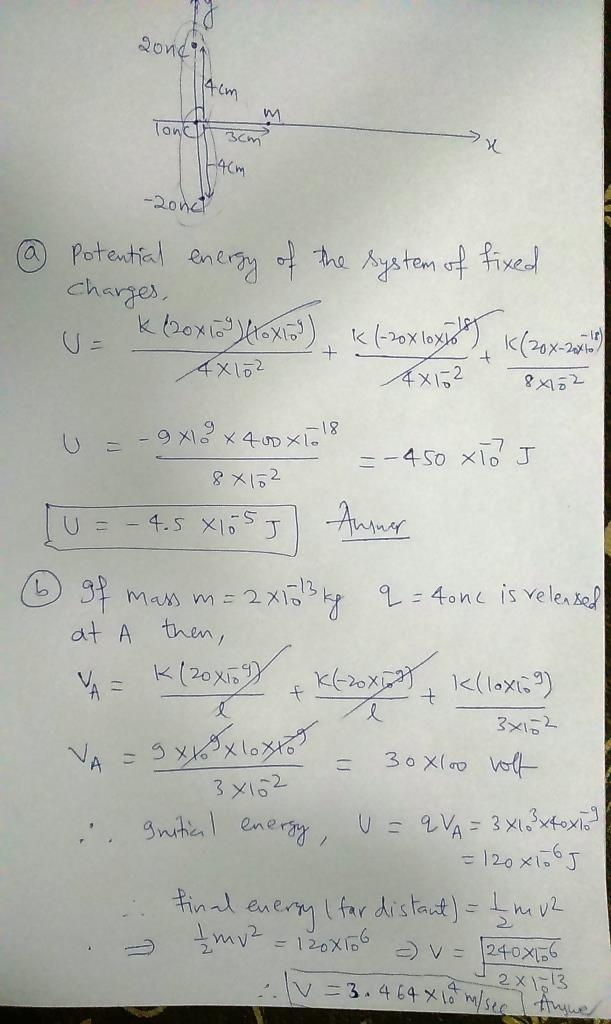Earn Coins

Coins can be redeemed for fabulous gifts.

Similar Homework Help Questions
• HELP PLEASE!!! If you do, I'll give u a good rating :)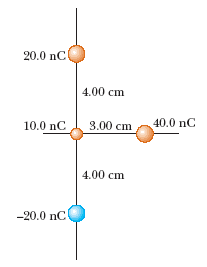Two particles, with charges of 20.0 nC and -20.0 nC, are placed atthe points with coordinates (0, 4.00 cm) and (0, -4.00 cm) as shownin Figure P20.18. A particle withcharge 10.0 nC is located at theorigin.Figure P20.18(a) Find the electric potential energy of theconfiguration of the three fixed charges.Your answer differs from the correct answerby 10% to 100%. J(b) A fourth particle, with a mass of 2.00 10-13 kgand a charge of 40.0 nC, is released fromrest at the point...

• Iwo particles, with charges of q1-90.U nC and q2 =-90.0 nC, are placed at the points...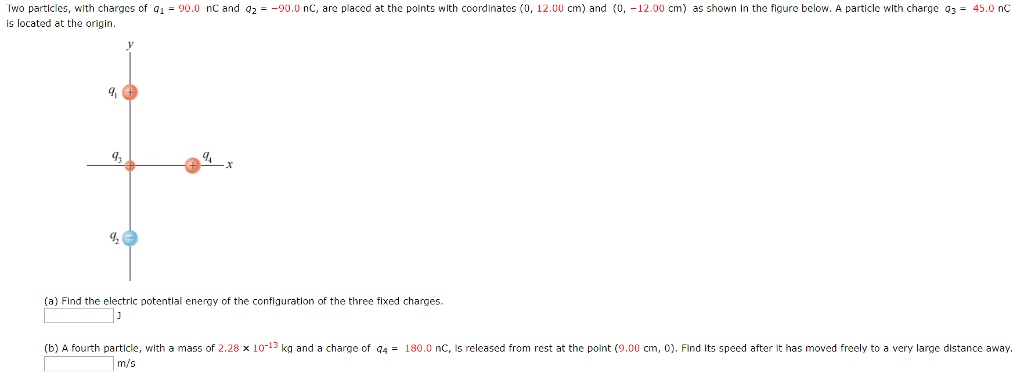Iwo particles, with charges of q1-90.U nC and q2 =-90.0 nC, are placed at the points with coordinates (0, 12.00 cm) and (0,-12.00 cm) as shown in the figure below. A particle with charge q3-45.0 nC is located at the origin. (a) Flnd the electric potentlal energy of the conflguratlon of the three fixed charges (b) A fourth particle, with a mass of 2.28 x 10-13 ka and a charge of q4 = 180.0 nC, is released from rest at...

• Electric potential due to point charges (second part)

[I already have part a) answered, just need part b) now] Two particles, with charges of 40.0 nC and -40.0 nC, are placed at thepoints in the xy plane with coordinates (0, 12.00) cm and (0, -12.00)cm . A particle with charge 20.0 nC is located at the origin. a) Find theelectric potential energy of the configuration of the three fixed charges.b) A fourth particle, with a mass of 2.00X10^-13kg and a charge of 40.0nC, is released from rest at...

• Point Charges- Electrical Potential Energy

Two particles, with charges of 20 nC and -20nC, are placed at the points with coordinates (0, 4 cm) and (0, -4 cm). A particle with charge 10 nC is located at theorigin.(a) Find the electric potential energy of the configuration of the three fixed charges.(b) A fourth particle, with a mass of 2.00*10^-13 kg and a charge of 40 nC is released from rest at the point (3 cm, 0). Find its speed after it has moved freely to...

• Three charged particles of 9 = 30.0 nC, 9, = -30.0 nc, and 97 = 15.0...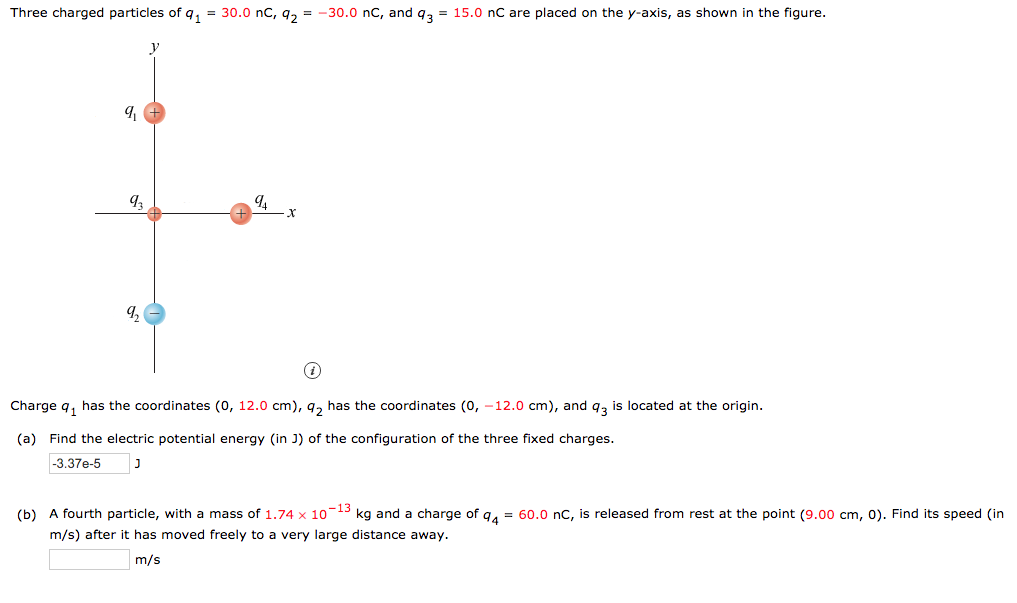Three charged particles of 9 = 30.0 nC, 9, = -30.0 nc, and 97 = 15.0 nC are placed on the y-axis, as shown in the figure. 42 9,- Charge q, has the coordinates (0, 12.0 cm), 9, has the coordinates (0, -12.0 cm), and q3 is located at the origin. (a) Find the electric potential energy in J) of the configuration of the three fixed charges. -3.37e-5 ] (b) A fourth particle, with a mass of 1.74 x 10-13...

• 6. 112 points | Previous Answers SerPSE9 25.P017 My Notes Ask Your Teacher Two particles, with...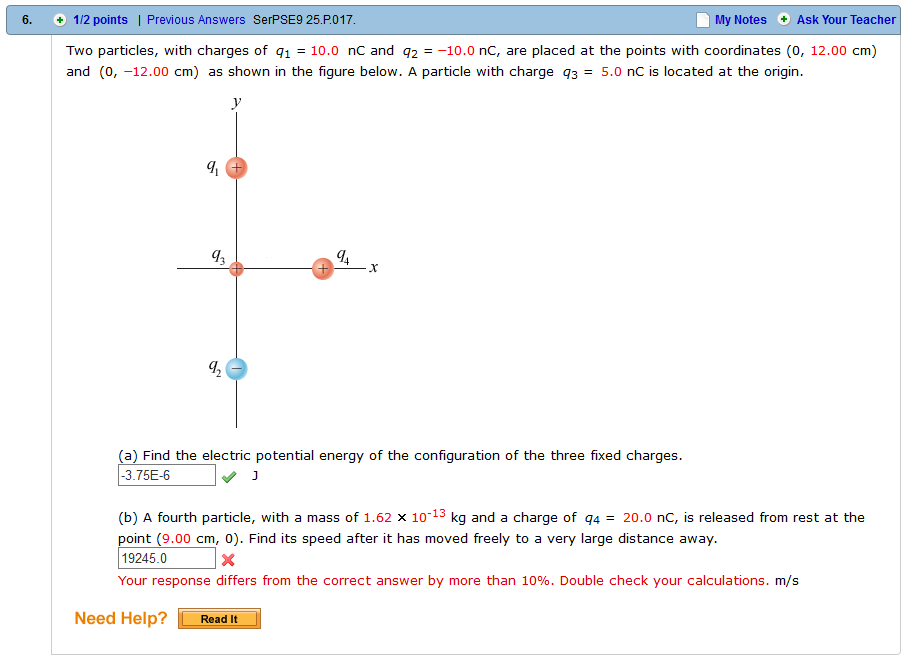6. 112 points | Previous Answers SerPSE9 25.P017 My Notes Ask Your Teacher Two particles, with charges of 10.0 nC and q210.0 nc, are placed at the points with coordinates (0, 12.00 cm) and (0, -12.00 cm) as shown in the figure below. A particle with charge q3-5.0 nC is located at the origin. q, q. (a) Find the electric potential energy of the configuration of the three fixed charges. 3.75E-6 (b) A fourth particle, with a mass of 1.62...

• Three charged particles of q1 = 90.0 nC, q,--90.0 nC, and q3 = 45.0 nC are...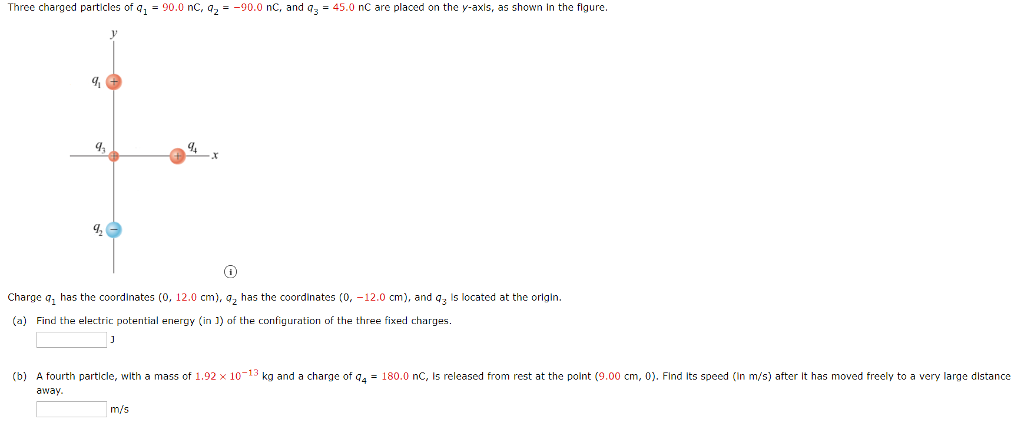Three charged particles of q1 = 90.0 nC, q,--90.0 nC, and q3 = 45.0 nC are placed on the y-axis, as shown in the figure g, I2 Charge a, has the coordlnates (0, 12.0 cm), a2 has the coordinates (0, -12.0 cm), and a3 Is located at the orlgin. (a) Find the electric potential energy (in 1) of the configuration of the three fixed charges. A fourth particle, with a mass of 1.92 × 10-13 kg and a charge of...

• Electric potential due to point charges

Two particles, with charges of 40.0 nC and -40.0 nC, are placed at thepoints in the xy plane with coordinates (0, 12.00) cm and (0, -12.00)cm . A particle with charge 20.0 nC is located at the origin. Find theelectric potential energy of the configuration of the three fixed charges.

• Two particles, with charges of 20 nC and 20 nC, are placed at the points with...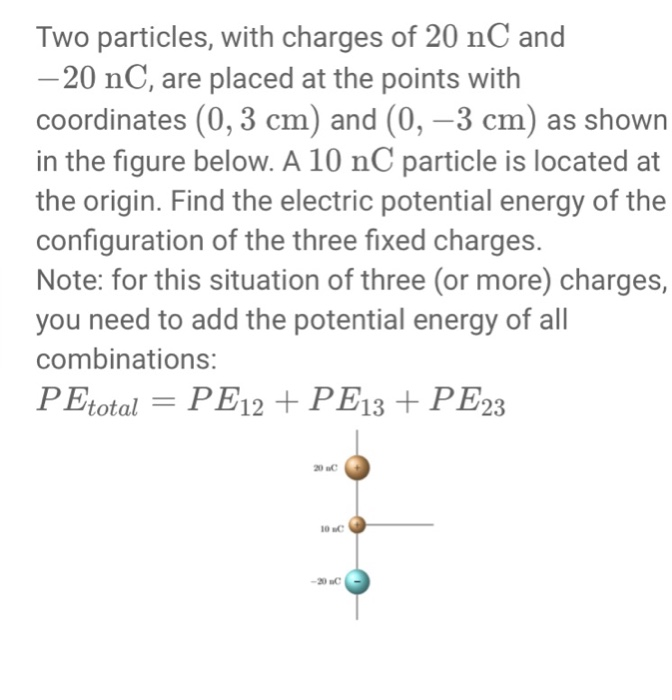Two particles, with charges of 20 nC and 20 nC, are placed at the points with coordinates (0, 3 cm) and (0,-3 cm) as shown in the figure below. A 10 nC particle is located at the origin. Find the electric potential energy of the configuration of the three fixed charges. Note: for this situation of three (or more) charges, you need to add the potential energy of all combinations: PEtotal-PE12+ PE13 + PE23

• Three point charges are placed on the x-y plane: a +40.0 nC charge at the origin,...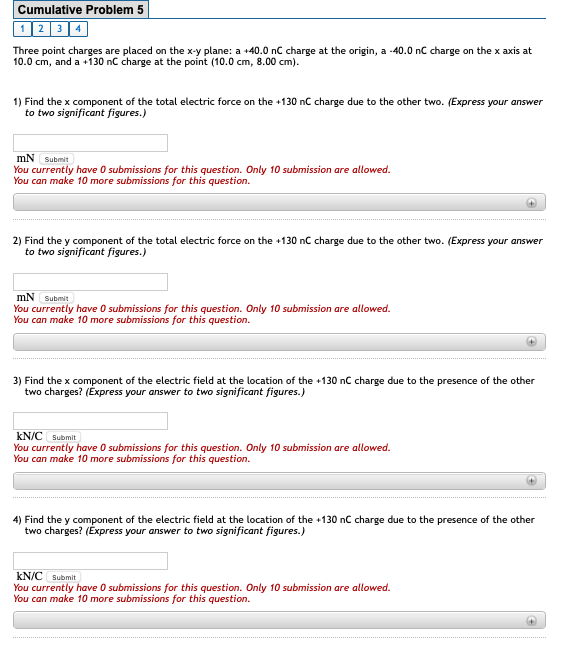Three point charges are placed on the x-y plane: a +40.0 nC charge at the origin, a -40.0 nC charge on the x axis at 10.0 cm, and a +130 nC charge at the point (10.0 cm, 8.00 cm).